# ISEE Lower Level Quantitative : How to find the decimal equivalent of a fraction

## Example Questions

← Previous 1 3 4 5 6

### Example Question #1 : How To Find The Decimal Equivalent Of A Fraction

Which fraction is the smallest?

Possible Answers: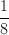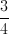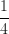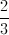Correct answer:Explanation:

Fractions get smaller as the numerator (top number) gets smaller, and the denominator (bottom number) gets bigger.has the smallest numerator AND the largest denominator of any of the fractions.

### Example Question #1 : Fractions

Convert 0.5 into a fraction.

Possible Answers: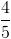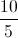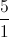Correct answer:Explanation:

If a number is one position to the right of the decimal, the the decimal is equal to that number divided by ten. 0.5 is equal to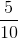.

We reduce the fraction to its simplest form. We divide the numerator by 5 and the denominator by 5.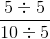### Example Question #1 : How To Find The Decimal Equivalent Of A Fraction

Convert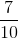into a decimal.

Possible Answers: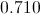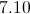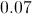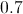Correct answer:Explanation:is also called "seven-tenths". The tenths spot is to the right of the decimal point.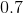is equal to seven tenths.

Ones: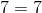Tenths: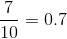Hundredths: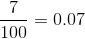Thousandths: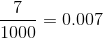### Example Question #1 : Fractions

Which is the smallest fraction?

Possible Answers: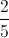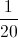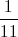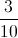Correct answer:Explanation: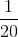is the smallest fraction because it has the biggest denominator (which means that all of the pieces it's divided up into are the smallest out of the choices). Also, only 1 of those pieces would be shaded, so it is the smallest out of the choices.

### Example Question #1 : Fractions

Find the decimal equivalent of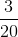.

Possible Answers: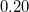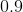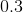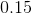Correct answer:Explanation:

To convert a fraction to a decimal, the denominator of the fraction must be 100.becomes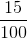. When the number is over one hundred, you can simply write it as a decimal, giving you 0.15.

### Example Question #6 : Fractions

Write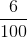as a decimal.

Possible Answers:Correct answer:Explanation:

The fraction is 6 hundredths, therefore the decimal must have the 6 in the hundredths place, or .06.

### Example Question #1 : How To Find The Decimal Equivalent Of A Fraction

What is the decimal equivalent to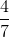, rounded to two decimal points?

Possible Answers: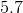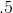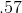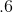Correct answer:Explanation:

To convert a fraction into a decimal, divide the numerator by the denominator.: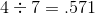This number has three digits of precision, but the question stem asks for two.is less than, so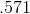rounds down to.

### Example Question #8 : Fractions

Which fraction is largest?

Possible Answers:Correct answer:Explanation:is equal to 50%, while all the other fractions are between 10% and 36%. A good rule of thumb is that the smaller the denominator, the larger the fraction's value.

### Example Question #1 : How To Find The Decimal Equivalent Of A Fraction

Which of these numbers is equal to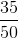?

Possible Answers: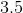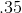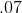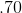Correct answer:Explanation:

To change a fraction into a decimal, divide the numerator by the denominator.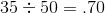### Example Question #1 : How To Find The Decimal Equivalent Of A Fraction

Which of these numbers is equal to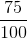?

Possible Answers: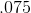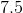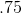Correct answer:Explanation:

To convert a fraction into a decimal, divide the numerator by the denominator.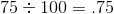← Previous 1 3 4 5 6

### All ISEE Lower Level Quantitative Resources